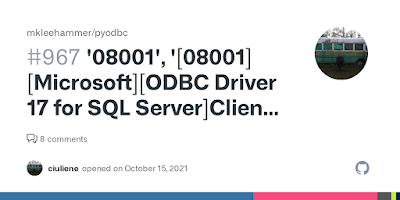## Saturday, 14 April 2012

### PHP Associative Arrays and Hashtable

Our today’s topic is how associative arrays are implemented in PHP. Let’s  discuss over it….

Basic Arrays in C

Let’s take an example of basic array in C where index or key is always an integer. It can’t be string. All the arrays start from index 0 and ends at index (n-1), where n is the number of elements in array. All the elements are stored in continuous memory locations. For example first element at memory location 0 and 10th  element on 9th location.  This is the basic array of C.

What is Hashtable?

A hash table or hash map is a data structure that uses a hash function to map identifying values, known as keys (e.g., a person's name), to their associated values (e.g., their telephone number). Thus, a hash table implements an associative array.  We will go into detail of this in next few minutes..

What is Hash Function?

The hash function is used to transform the key into the index (the hash) of an array element (the slot or bucket) where the corresponding value is to be sought. There is no magic formula for the creation of the hash function. It can be any mathematical transformation that produces a relatively random and unique distribution of values within the address space of the storage.

PHP and Hashtable (an implementation of a map):

In PHP associative arrays can be implemented using hash-maps or b-trees. This has important implications on the performance characteristics of associative arrays and how they should be used; e.g. b-trees are slow to insert but handle collisions better than hash maps.  Hashmaps are faster if there are no collisions, but are slower to retrieve when there are collisions. In PHP associative arrays are actually implemented using hashtables with ordered map or you can say hashmaps.  Following are the actual functions of PHP written in C to implement hashtable. Check here.

struct _hashtable;
typedef struct bucket {
ulong h;                                                                                  /* Used for numeric indexing */
uint nKeyLength;
void *pData;
void *pDataPtr;
struct bucket *pListNext;
struct bucket *pListLast;
struct bucket *pNext;
struct bucket *pLast;
const char *arKey;
} Bucket;

typedef struct _hashtable {
uint nTableSize;
uint nNumOfElements;
ulong nNextFreeElement;
Bucket *pInternalPointer;      /* Used for element traversal */
Bucket *pListTail;
Bucket **arBuckets;
dtor_func_t pDestructor;
zend_bool persistent;
unsigned char nApplyCount;
zend_bool bApplyProtection;
#if ZEND_DEBUG
int inconsistent;
#endif
} HashTable;

In PHP, associative array key(string) is first converted into index using hash function and then that value is stored at that particular index location. Here is again a problem. Hash function returns an integer that is system dependent (e.g 32bit or 64 bit).

For example say, a system with 32 bit OS, against a key named ‘dirtyhandsphp’ hash function returns :
00000000010111111010101010111000

It’s decimal value is 6269624.

This is a huge value and can’t represent the location with hashtable size of 2048(say). So here PHP use one more trick. It throws some digits away by using table mask.

(ht)->nTableMask = (ht)->nTableSize - 1;

It’s one less than the size of hash table. Because if table size is 2048(210) then maximum index could be 2047 starting from 0.

PHP performs & operation between both of them

00000000010111111010101010111000
&    00000000000000000000001111111111
______________________________________________________
00000000000000000000001010111000 = 676

So the element would be stored at 676th location.

Collision: Implementing hash table in this way produces some problems called collision. For example hash function can generate same integer value for two different strings or you can say for associative array keys.  Two locations may refer to same index. Such situation is called collision. In PHP to solve such type of problem, it uses linked list. So if same index is generated then it would stored at different location but would linked to the same index generated using hash function.

To read or access the element again same process will follow :

1) Hash is calculated
2) Use table mask
3) Locate the memory address and
4) Get the data or if required traverse the linked list to find the element we needed.

Thanks !!!!!!!!!!! Enjoy Programming :)